Courses

#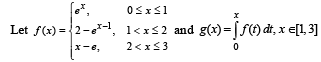then g(x) hasa)local maxima at x = 1 + ln 2 and local minima at x = eb)local maxima at x = 1 and local minima at x = 2c)no local maximad)no local minimaCorrect answer is option 'A,B'. Can you explain this answer? Related Test: Multiple Correct MCQ Of Applications Of Derivatives, Past Year Questions JEE Advance, Class 12, Maths

## JEE QuestionChander Bhan Oct 15, 2020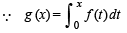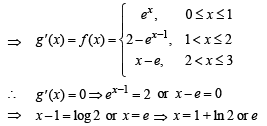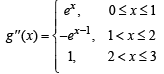∴ g ''(1 + ln 2) =-2 and g ''(e) = 1⇒ g (x) has local max. at x = 1 + ln 2 and local min. at x = e.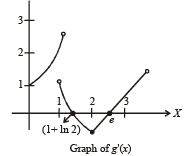Also graph of g '(x) suggests, g (x) has local max. at x = 1 and local min. at x = 2Ashish Khanda Jan 22, 2021
Correct is A and B

This discussion on then g(x) hasa)local maxima at x = 1 + ln 2 and local minima at x = eb)local maxima at x = 1 and local minima at x = 2c)no local maximad)no local minimaCorrect answer is option 'A,B'. Can you explain this answer? is done on EduRev Study Group by JEE Students. The Questions and Answers of then g(x) hasa)local maxima at x = 1 + ln 2 and local minima at x = eb)local maxima at x = 1 and local minima at x = 2c)no local maximad)no local minimaCorrect answer is option 'A,B'. Can you explain this answer? are solved by group of students and teacher of JEE, which is also the largest student community of JEE. If the answer is not available please wait for a while and a community member will probably answer this soon. You can study other questions, MCQs, videos and tests for JEE on EduRev and even discuss your questions like then g(x) hasa)local maxima at x = 1 + ln 2 and local minima at x = eb)local maxima at x = 1 and local minima at x = 2c)no local maximad)no local minimaCorrect answer is option 'A,B'. Can you explain this answer? over here on EduRev! Apart from being the largest JEE community, EduRev has the largest solved Question bank for JEE.